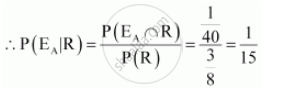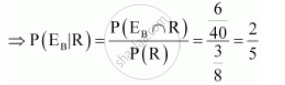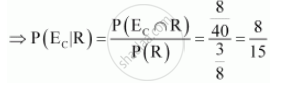Share

# Suppose We Have Four Boxes. A, B, C and D Containing Coloured Marbles as Given Below: One of the Boxes Has Been Selected at Random and a Single Marble is Drawn from It. If the Marble is Red, What is the Probability that It Was Drawn from Box A?, Box B?, Box C? - CBSE (Commerce) Class 12 - Mathematics

#### Question

Suppose we have four boxes. A, B, C and D containing coloured marbles as given below:

 Box Marble colour Red White Black A 1 6 3 B 6 2 2 C 8 1 1 D 0 6 4

One of the boxes has been selected at random and a single marble is drawn from it. If the marble is red, what is the probability that it was drawn from box A?, box B?, box C?

#### Solution

Let R be the event of drawing the red marble.

Let EA, EB, and EC respectively denote the events of selecting the box A, B, and C.

Total number of marbles = 40

Number of red marbles = 15

:.P(R) = 15/40 =3/8

Probability of drawing the red marble from box A is given by P (EA|R).Probability that the red marble is from box B is P (EB|R).Probability that the red marble is from box C is P (EC|R).Is there an error in this question or solution?

#### Video TutorialsVIEW ALL 

Solution Suppose We Have Four Boxes. A, B, C and D Containing Coloured Marbles as Given Below: One of the Boxes Has Been Selected at Random and a Single Marble is Drawn from It. If the Marble is Red, What is the Probability that It Was Drawn from Box A?, Box B?, Box C? Concept: Conditional Probability.
S